Anzeige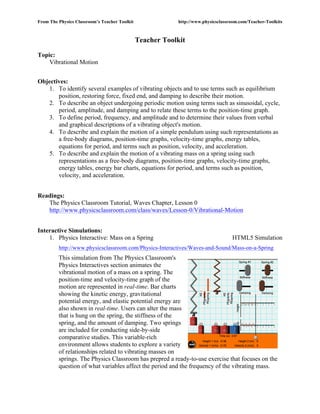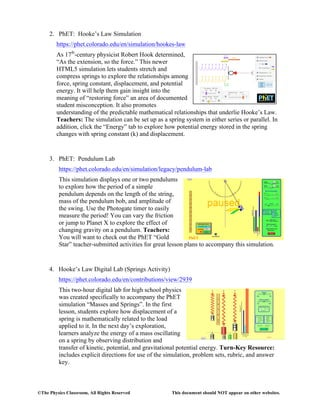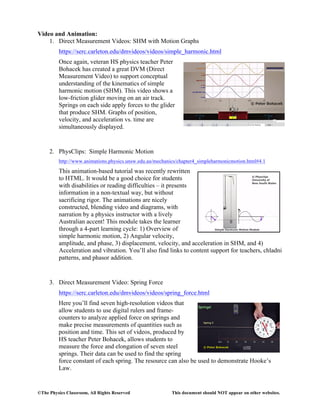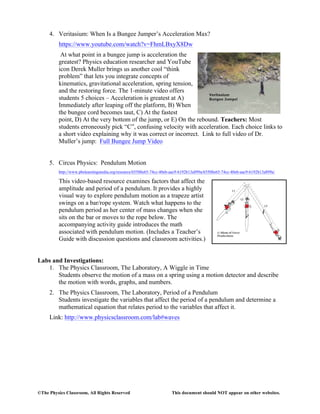Anzeige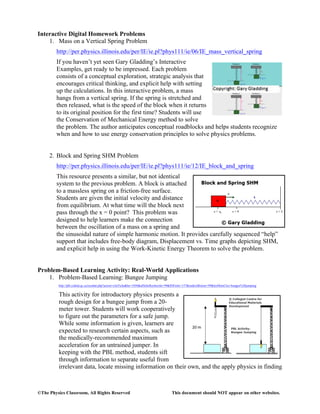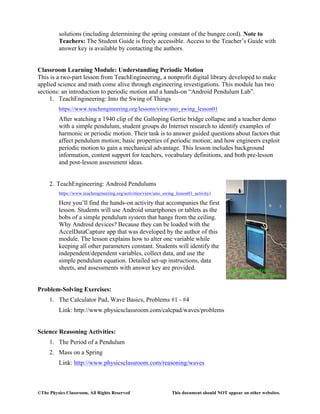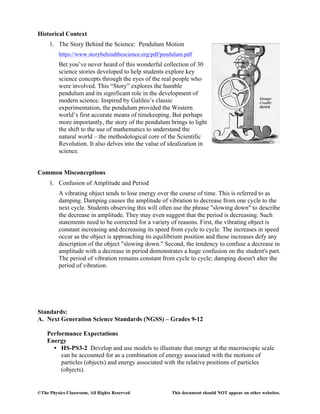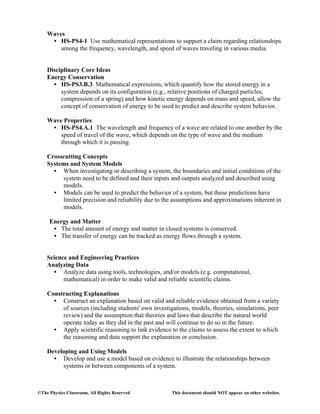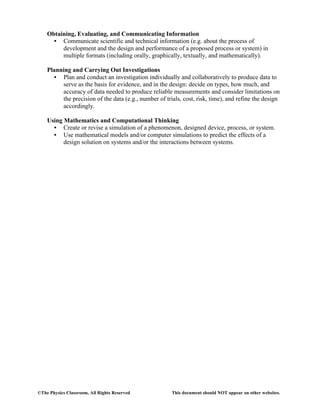Nächste SlideShare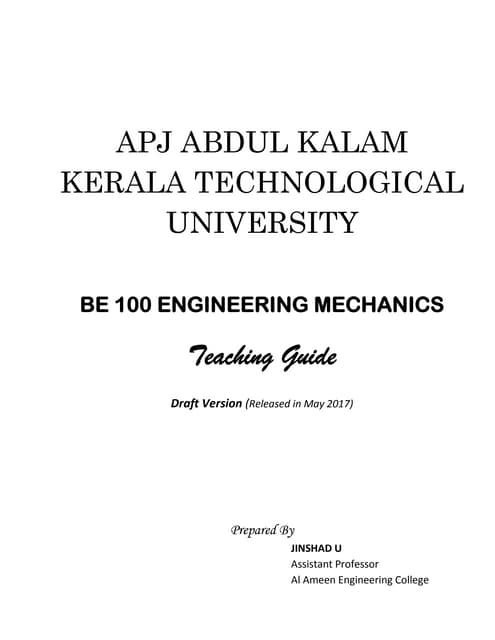KTU BE 100 Engineering Mechanics
1 von 9
Anzeige

### VibrationalMotion (1).pdf

1. From The Physics Classroom’s Teacher Toolkit http://www.physicsclassroom.com/Teacher-Toolkits Teacher Toolkit Topic: Vibrational Motion Objectives: 1. To identify several examples of vibrating objects and to use terms such as equilibrium position, restoring force, fixed end, and damping to describe their motion. 2. To describe an object undergoing periodic motion using terms such as sinusoidal, cycle, period, amplitude, and damping and to relate these terms to the position-time graph. 3. To define period, frequency, and amplitude and to determine their values from verbal and graphical descriptions of a vibrating object's motion. 4. To describe and explain the motion of a simple pendulum using such representations as a free-body diagrams, position-time graphs, velocity-time graphs, energy tables, equations for period, and terms such as position, velocity, and acceleration. 5. To describe and explain the motion of a vibrating mass on a spring using such representations as a free-body diagrams, position-time graphs, velocity-time graphs, energy tables, energy bar charts, equations for period, and terms such as position, velocity, and acceleration. Readings: The Physics Classroom Tutorial, Waves Chapter, Lesson 0 http://www.physicsclassroom.com/class/waves/Lesson-0/Vibrational-Motion Interactive Simulations: 1. Physics Interactive: Mass on a Spring HTML5 Simulation http://www.physicsclassroom.com/Physics-Interactives/Waves-and-Sound/Mass-on-a-Spring This simulation from The Physics Classroom's Physics Interactives section animates the vibrational motion of a mass on a spring. The position-time and velocity-time graph of the motion are represented in real-time. Bar charts showing the kinetic energy, gravitational potential energy, and elastic potential energy are also shown in real-time. Users can alter the mass that is hung on the spring, the stiffness of the spring, and the amount of damping. Two springs are included for conducting side-by-side comparative studies. This variable-rich environment allows students to explore a variety of relationships related to vibrating masses on springs. The Physics Classroom has prepred a ready-to-use exercise that focuses on the question of what variables affect the period and the frequency of the vibrating mass.